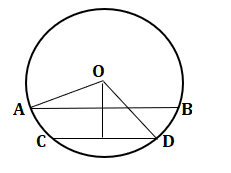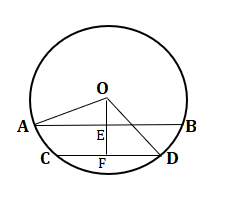GMAT Question of the Day - Daily to your Mailbox; hard ones only

 It is currently 20 Oct 2019, 14:34GMAT Club Daily Prep

Thank you for using the timer - this advanced tool can estimate your performance and suggest more practice questions. We have subscribed you to Daily Prep Questions via email.

Customized
for You

we will pick new questions that match your level based on your Timer History

Track

every week, we’ll send you an estimated GMAT score based on your performance

Practice
Pays

we will pick new questions that match your level based on your Timer History

Not interested in getting valuable practice questions and articles delivered to your email? No problem, unsubscribe here.AB and CD are two parallel chords of a circle, with center O .........

 new topic post reply Question banks Downloads My Bookmarks Reviews Important topics
Author Message
TAGS:

Hide Tags

e-GMAT RepresentativeV
Joined: 04 Jan 2015
Posts: 3078
AB and CD are two parallel chords of a circle, with center O .........  [#permalink]

Show Tags

1
200:00

Difficulty:25% (medium)

Question Stats:77% (02:15) correct23% (02:47) wrongbased on 93 sessions

HideShow timer StatisticsAB and CD are two parallel chords of a circle, with center O, such that AB = 12 and CD = 6. If the diameter of the circle is 6√5, then find the distance between the two chords?

A. √3
B. 3
C. √5
D. 2√3
E. 6

_________________
NUS School ModeratorV
Joined: 18 Jul 2018
Posts: 1021
Location: India
Concentration: Finance, Marketing
WE: Engineering (Energy and Utilities)
AB and CD are two parallel chords of a circle, with center O .........  [#permalink]

Show Tags

OA = radius = $$6\sqrt{5}/2$$ = $$3\sqrt{5}$$ = OD

From the attached below image. AE = 6.
CF = 3.
Then OE as per Pythagorean theorem gives $$\sqrt{45-36}$$ = 3.
OF = $$\sqrt{45-9}$$= 6.

OF = OE + EF
EF = 6-3 = 3.

B is the answer.
Attachmentsps1.png [ 7.86 KiB | Viewed 1232 times ]

_________________
Press +1 Kudos If my post helps!
e-GMAT RepresentativeV
Joined: 04 Jan 2015
Posts: 3078
AB and CD are two parallel chords of a circle, with center O .........  [#permalink]

Show Tags

Solution

Given:

We are given that,
• AB and CD are two parallel chords of a circle
• O is the center of the circle
• AB = 12 and CD = 6
• Diameter of the circle = 6√5

To find:
• The distance between the two chords, AB and CD

Approach and Working:From the above figure, we can infer that we need to find the value of EF

In triangle, OAE, we know that,
• $$AE = \frac{AB}{2} = \frac{12}{2} = 6$$
• OA = radius of the circle =$$\frac{6√5}{2} = 3√5$$
• $$∠OEA = 90^o$$

So, we can write, $$OA^2 = OE^2 + AE^2$$
• Implies, $$(3√5)^2 = OE^2 + 6^2$$
• $$OE^2 = 45 – 36 = 9$$
• Thus, OE = √9 = 3

Similarly, in triangle DOF, we ca say that
• $$OD^2 = OF^2 + FD^2$$
o $$(3√5)^2 = OF^2 + (\frac{6}{2})^2$$
o $$OF^2 = 45 – 9 = 36$$

• Thus, OF = √36 = 6

Therefore, EF = OF = OE = 6 – 3 = 3

Hence the correct answer is Option B.

_________________
Senior ManagerG
Joined: 13 Feb 2018
Posts: 450
GMAT 1: 640 Q48 V28Re: AB and CD are two parallel chords of a circle, with center O .........  [#permalink]

Show Tags

EgmatQuantExpert

Please explain a bit more why AE=EB

Regards
L
e-GMAT RepresentativeV
Joined: 04 Jan 2015
Posts: 3078
Re: AB and CD are two parallel chords of a circle, with center O .........  [#permalink]

Show Tags

LevanKhukhunashvili wrote:
EgmatQuantExpert

Please explain a bit more why AE=EB

Regards
L

Hi,

If you see the two triangles AOE and EOB, both are congruent triangles.
AO = OB = radius,
OE is a common side, and
angle OEA = angle OEB = 90 degrees

Thus, the length of the third sides must be equal, that is, AE = EB

Regards,
_________________Re: AB and CD are two parallel chords of a circle, with center O .........   [#permalink] 22 Jan 2019, 23:38
Display posts from previous: Sort by

AB and CD are two parallel chords of a circle, with center O .........

 new topic post reply Question banks Downloads My Bookmarks Reviews Important topics

 Powered by phpBB © phpBB Group | Emoji artwork provided by EmojiOne